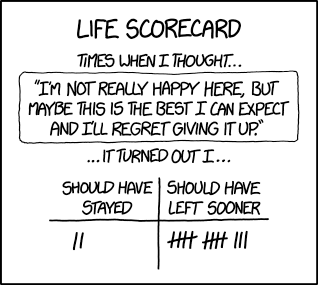## going to war [a riddle]On the Riddler this week, a seemingly obvious riddle:

A game consists of Alice and Bob, each with a $1 bill, receiving a U(0,1) strength each, unknown to the other, and deciding or not to bet on this strength being larger than the opponent’s. If no player bets, they both keep their$1 bill. Else, the winner leaves with both bills. Find the optimal strategy.

As often when “optimality” is mentioned, the riddle is unclear because, when looking at the problem from a decision-theoretic perspective, the loss function of each player is not defined in the question. But the St. Petersburg paradox shows the type of loss clearly matters and the utility of money is anything but linear for large values, as explained by Daniel Bernoulli in 1738 (and later analysed by Laplace in his Essai Philosophique).  Let us assume therefore that both players live in circumstances when losing or winning \$1 makes little difference, hence when the utility is linear. A loss function attached to the experiment for Alice [and a corresponding utility function for Bob] could then be a function of (a,b), the result of both Uniform draws, and of the decisions δ¹ and δ² of both players as being zero if δ¹=δ²=0 and$L(a,b,\delta^1,\delta^2)=\begin{cases}0&\text{if }\delta^1=\delta^2=0\\\mathbb{I}(ab)&\text{else}\\\end{cases}$

Considering this loss function, Alice aims at minimising the expected loss by her choice of δ¹, equal to zero or one, expected loss that hence depends  on the unknown and simultaneous decision of Bob. If for instance Alice assumes Bob takes the decision to compete when observing an outcome b larger than a certain bound α, her decision is based on the comparison of (when B is Uniform (0,1))$\mathbb{P}(a\alpha)-\mathbb{P}(a>B,B>\alpha)=2(1-a\vee\alpha)-(1-\alpha)$

(if δ¹=0) and of 1-2a (if δ¹=1). Comparing both expected losses leads to Alice competing (δ¹=1) when a>α/2.

However, there is no reason Alice should know the value of α when playing the (single) game and so she may think that Bob will follow the same reasoning, leading him to choosing a new bound of α/4, and, by iterating the thought process, down all the way to α=0!  So this modelling leads to always play the game, with each player having a ½ probability to win… Alternatively, Alice may set a prior on α, which leads to another bound on a for playing or not the game. Which in itself is not satisfactory either. (The published solution is following the above argument. Except for posting the maths expressions.)

This site uses Akismet to reduce spam. Learn how your comment data is processed.